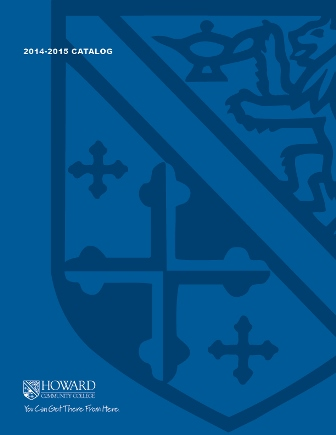2017-2018 College Catalog# MATH 141 College Algebra

Topics include functions, mathematics of finance, matrices, and linear programming. Emphasis will be placed on solving problems algebraically and modeling applications involving global awareness and financial literacy.  Appropriate technological tools used in business and the social sciences will also be introduced. All sections require the use of the interactive computer program that comes with the software code and a graphing calculator (TI-84 recommended. TI-89 not permitted).

3

### Prerequisite

MATH 070 or appropriate score on mathematics placement test

4 hours weekly

### Course Objectives

1. 1. Communicate college-level mathematical concepts related to functions, matrices, and linear
programming using appropriate symbols, notation, and language.
2. 2. Construct graphs and equations of polynomial, rational, exponential, and logarithm functions and describe the characteristics of the functions.
3. 3. Identify and graph shifts, reflections, and transformations of functions.
4. 4. Model and solve applied problems involving exponential functions including models of exponential growth that appear in finance and population problems.
5. 5. Use compound and continuous interest formulas to solve problems involving loans and investments, such as total interest paid, present value, future value, growth rate or time period, and the annual percentage yield.
6. 6. Analyze annuities to calculate payments, total interest, present value and future value, and to construct an amortization schedule.
7. 7. Perform matrix operations and solve linear systems of equations and applications using matrices.
8. 8. Solve linear programming problems using the graphing and simplex method.
9. 9. Model applications related to real-world issues including global awareness, sustainability, and financial literacy.
10. 10. Analyze and evaluate solutions to equations algebraically and graphically for the reasonableness of the solution.
11. 11. Identify and implement appropriate technologies to efficiently complete tasks that involve the solving of cross-discipline, mathematically appropriate problems and creating new works to communicate the processes used and solution.

### Course Objectives

1. Communicate college-level mathematical concepts related to functions, matrices, and linear
programming using appropriate symbols, notation, and language.

2. Construct graphs and equations of polynomial, rational, exponential, and logarithm functions and describe the characteristics of the functions.

3. Identify and graph shifts, reflections, and transformations of functions.

4. Model and solve applied problems involving exponential functions including models of exponential growth that appear in finance and population problems.

5. Use compound and continuous interest formulas to solve problems involving loans and investments, such as total interest paid, present value, future value, growth rate or time period, and the annual percentage yield.

6. Analyze annuities to calculate payments, total interest, present value and future value, and to construct an amortization schedule.

7. Perform matrix operations and solve linear systems of equations and applications using matrices.

8. Solve linear programming problems using the graphing and simplex method.

9. Model applications related to real-world issues including global awareness, sustainability, and financial literacy.

10. Analyze and evaluate solutions to equations algebraically and graphically for the reasonableness of the solution.

11. Identify and implement appropriate technologies to efficiently complete tasks that involve the solving of cross-discipline, mathematically appropriate problems and creating new works to communicate the processes used and solution.